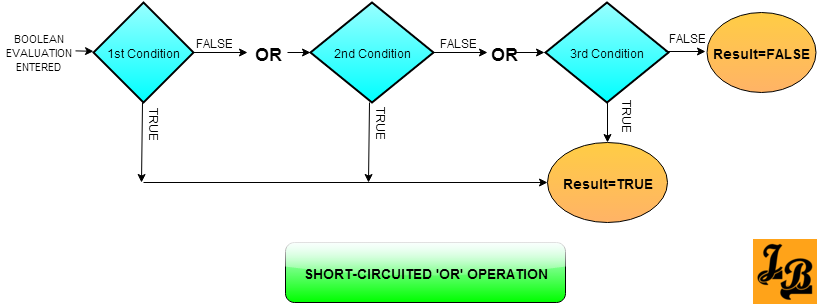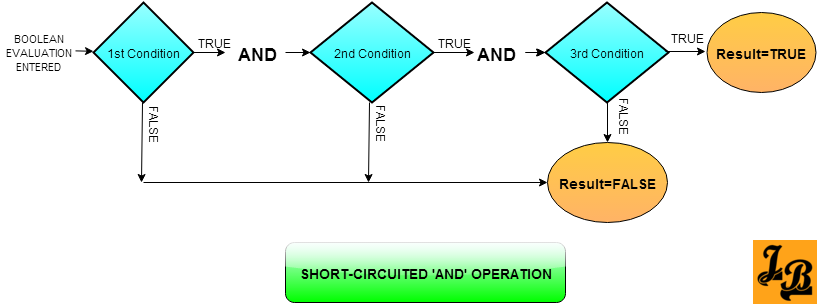Short-Circuiting Or Short-Circuits in Boolean Evaluations in Programming and Java
This article explains the concept of Short-Circuiting Or Short-Circuits in Boolean Evaluations and then takes a look at how it is used in Java.
What are Short Circuit Evaluations In boolean logic whenever we have multiple conditions in sequence i.e. conditions getting checked are ANDed or ORed one after the other, then short circuit evaluation ignores checking the remaining conditions once it has determined that the result of the remaining conditions is not going to affect the already evaluated result. This leads to efficient processing as unnecessary condition checks are avoided.
Note - Short Circuit evaluations are also referred to as minimal evaluations or McCarthy evaluations after John McCarthy who was the creator of Lisp. Examples of Short Circuit Evaluations Example 1: Conditions are ORed in sequence - Lets take the scenario of multiple conditions being ORed together in a boolean valued expression. The evaluation of these conditions starts from the left. On the first condition being evaluated to true for any of the conditions, the remaining conditions need not be evaluated, i.e. the boolean expression evaluation will be short-circuited, and result of the evaluation will be true.This is because if all the conditions are ORed together and atleast one of the conditions is true, then the boolean expression will evaluate to true.

Represented as a flowchart the ORed conditions' check with short-circuit evaluation would look like this -Explanation of the above flowchart
• 3 conditions are ORed in a sequence
• There are short circuit paths below condition-1 & condition-2 marked with TRUE which directly lead to result being determined to TRUE.
• If condition-1 is TRUE then short-circuit path to result being TRUE is taken. Condition-2 & condition-3 are not evaluated and result is determined as TRUE.
• In case condition-1 is FALSE, and then condition-2 is found to be TRUE then short-circuit path to result being TRUE is taken. In this case condition-3 is not evaluated and result is determined as TRUE.
Example 2: Conditions are ANDed in sequence - In this scenario, similar to the ORed scenario in example 1, the 3 conditions to be evaluated are ANDed in a sequence. The conditions are evaluated from left to right. On any condition being found to be FALSE, the boolean evaluation is short-circuited to the result FALSE as shown in the flowchart below -Explanation of the above flowchart
• 3 conditions are ANDed in a sequence
• There are short circuit paths below condition-1 & condition-2 marked with FALSE which directly lead to result being determined to FALSE.
• If condition-1 is FALSE then short-circuit path to result being FALSE is taken. Condition-2 & condition-3 are not evaluated and result is determined as FALSE.
• In case condition-1 is TRUE, and then condition-2 is found to be FALSE then short-circuit path to result being FALSE is taken. In this case condition-3 is not evaluated and result is determined as FALSE.
Short-Circuit evaluations in Java Java supports two short-circuit operators - &&(AND) and ||(OR). Lets see few examples of short-circuit evaluations in Java -
Example 1: (2==2) ||(1==1)||(2=3)
This expression evaluates to TRUE. Once 2==2 is evaluated to TRUE, 1==1 and 2==3 are not evaluated as any boolean value ORed with TRUE from the first condition has to be TRUE.

Example 2: (2==2) && (1==2) && (3=3)
This expression evaluates to FALSE. First 2==2 is evaluated to TRUE, then 1==2 is evaluated which turns out to be FALSE. At this point the boolean expression evaluation is short-circuited to result being FALSE. Remaining 3==3 is not evaluated as any boolean value ANDed with FALSE obtained from second condition(1==2) will be FALSE.
Disadvantages of Short-Circuiting Although Short-Circuits have the obvious advantage of efficient processing because it skips unnecessary condition evaluations, there are some disadvantages of this approach as well -
Disadvantage 1: Any logic which was expected to be executed as part of the conditions which are bypassed will not be executed. I.e. say we have Condition-1 OR Condition-2 where condition-1 is evaluated to TRUE. So evaluation is short-circuited and condition-2 is not evaluated. By in this case, condition-2 was a method call which was supposed to complete a step in processing. In not evaluating condition-2 we are not invoking the said method and missing a part of execution.
Note - Java has two boolean operators &(AND) and |(OR). These boolean operators are not short-circuited. Using such operators Java programmers can avoid this disadvantage.

Disadvantage 2: Code execution becomes less efficient with short-circuited execution paths because in some compilers the new checks for short-circuits are extra execution cycles in themselves. Also, branch prediction becomes inefficient in some modern processors when short-circuiting is used.